## Revision

You should not use a calculator for any of the questions in this section. Do show your steps of working.

### Working with whole numbers

1. Make the number sentences below true. Into the first block in each question, write a multiplication or division sign. Into the second block, write either 10, 100 or 1 000.

1. 8 ☐ ☐ = 800

2. 740 000 ☐ ☐= 740

2. Circle all the numbers given below that will round off to 60 000.

62 495; 54 498 ; 65 000; 56 002 ; 67 024

3. Calculate the following:

1. $$274 561 + 367 238$$

2. $$4 672 - 3 937$$

3. $$3 458 \times 43$$

4. $$6 624 \div 18$$

4. Write the missing numbers in the blocks.

1. 8; 15; 22; 29; ☐

2.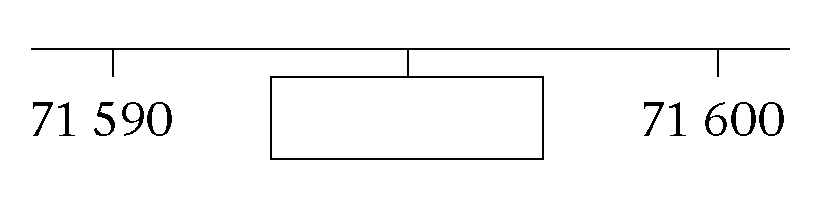5. Tumi makes a sequence of numbers using the following rule: "Take half the previous number and then add 12." Write the next three numbers in the sequence:

56; 40; 32;

6. Two three-digit numbers are added together as shown, and produce a three-digit answer - but some of the digits are missing. Fill in the missing digits so as to make the calculation correct.

59 ☐+ 3 ☐9=☐53

7. Ismail has the following numbers:

71; 72; 73; 74; 75; 76; 77; 78; 79; 80

He wishes to sort them by placing them in the sorting diagram below. Help Ismail by placing the numbers in the correct blocks.

 Prime number Not a prime number Multiple of 4 Not a multiple of 4
8. Write down, using only numbers from the cloud: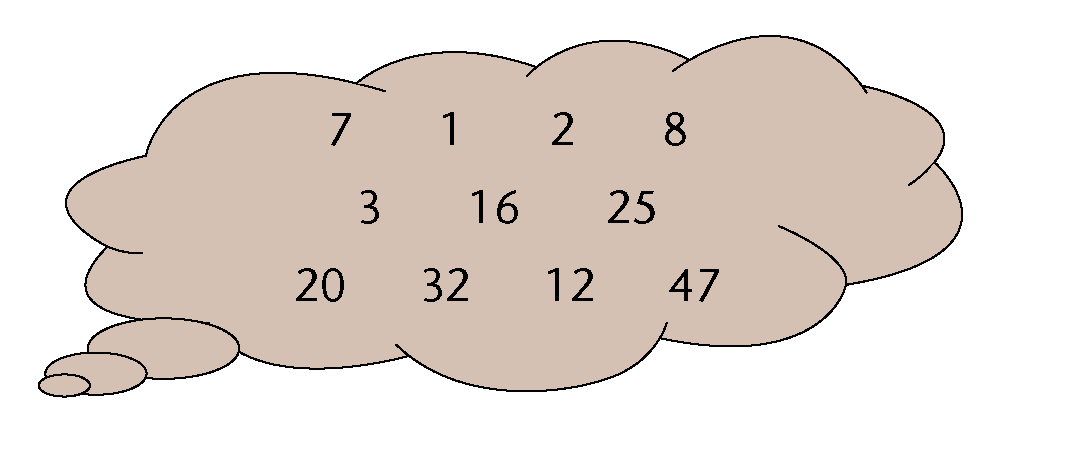1. All the prime numbers

2. All the square numbers

3. All the cube numbers

4. All the multiples of 8

5. All the factors of 8

9. Teacher Ramushwana states:

"Every even number (greater than or equal to 6) can be written as the sum of a

pair of odd prime numbers, for example $$10 = 3 + 7$$."

1. Write down two pairs of odd prime numbers that each sum to 20.

2. Choose any even number greater than 30 and write it as a sum of two odd prime numbers.

1. Write the following as a product of prime factors:

1. 576

2. 600

2. Find both the (i) HCF and (ii) LCM of 576 and 600.

1. HCF

10. How many hours will it take the Adams family to reach their holiday destination if it is 495 km away and they travel at an average speed of 110 km/h?

11. Graeme, Thuli and Andile have worked as a team over the holidays, mowing the lawns of their neighbours. They collected a total of R1 200 and now need to share it. They agree that as they didn't all work an equal amount, the money should be shared between Graeme, Thuli and Andile in the ratio 4 : 6 : 5. How much money will Thuli receive?

12. Mr Khumalo decides to try to make some money buying and selling used furniture. He has R6 000 in his bank account, and uses some of the money to buy an old bed base and mattress for R800, a dresser for R2 500, two lockers for R300 each, and a washing machine for R900.

1. How much is left in his bank account after these purchases?

2. Suppose that he sells the bed base and mattress for R980, the dresser for R2 950, and both lockers for a total of R750. Nobody seems to want his washing machine though. At this stage he has made a loss. What is the value of the loss?

3. How much does he need to sell the washing machine for to have an overall profit of R1 000?

13. Mrs Steyn takes out a loan of R55 000 from Fidelity Bank. The bank charges simple interest of R500 per month. How much money will Mrs Steyn owe after $$1\frac{1}{2}$$ years?

14. John earns R480 on a Saturday. He works from 08:00 to 14:00. Calculate his hourly rate.

### Exponents

1. Calculate.

1. $$12 \times 12$$

2. $$8 \times 8$$

3. $$7 \times 7 \times 7$$

4. $$3 \times 3 \times 3$$

5. $$6 \times 6 \times 6$$

6. $$13 \times 13$$

2. Explain the difference between $$4 \times 3$$ and $$4^3$$.

3. Write $$5^5$$ in expanded form.

4. Write the following in exponential form:

1. $$2 \times 2 \times 2$$

2. $$3 \times 3 \times 3 \times 3 \times 3 \times 3 \times 3 \times 3$$

1. 81

2. 10 000

6. Complete:

1. 5 squared is
2. 5 cubed is

7. Calculate:

1. $$7^2$$

2. $$15^2$$

3. $$3^2 \times 4^2$$

4. $$\sqrt{16}$$

1. The number 64 can be written both as a square and a cube.

2. $$2^5$$ is smaller than $$30^1$$.

1. $$\sqrt{216}$$

2. $$\sqrt{8}$$

3. $$\sqrt{125}$$
4. $$\sqrt{27}$$

10. Determine the value of each of the following:

1. $$3^2-2^3$$

2. $$4(10 - 1^{100})$$

3. $$(8-2)^2$$

4. $$\sqrt{4} \times \sqrt{81}$$

5. $$(\sqrt{58})^2$$
6. $$\sqrt{27} \div \sqrt{9}$$

7. $$10 \times \sqrt{81}$$

8. $$\sqrt{2 \times 32}$$
11. $$13=169; 14^2 =196; 15^2=225; 16^2=256; 7^3=343; 8^3=512; 3^3=27$$

Use these facts to calculate the value of each of the following:

1. $$\sqrt{196}-\sqrt{512}$$

2. $$\sqrt{169 \times 225}$$

3. $$\frac{\sqrt{196}}{\sqrt{343}}$$
4. $$\frac{14^2- 13^2}{3^3}$$

12. If $$56^3= 175 616$$, write down the value of $$\sqrt{175 616}$$.

### Geometry of straight lines

1. Consider the grid shown alongside.

1. Is PS a line, ray or line segment?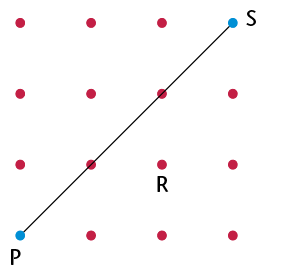2. Draw on the grid a line segment through R that will be perpendicular to PS. Label it TU.

3. Draw on the grid a line that is parallel to PS. Label it WX.

2. Provide the correct name for each of the geometric features AB and CD, shown on the diagram: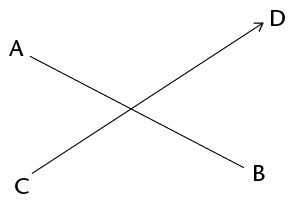AB:

CD:

3. There is a geometric relationship between line segments PR and QS shown in the diagram. Describe the relationship by adding the correct word on the dotted line: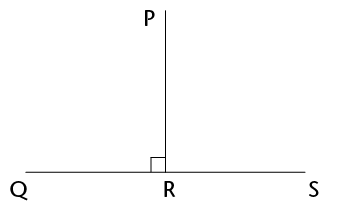PR is ____________ QS.

4. Draw a ray and a line that will never meet.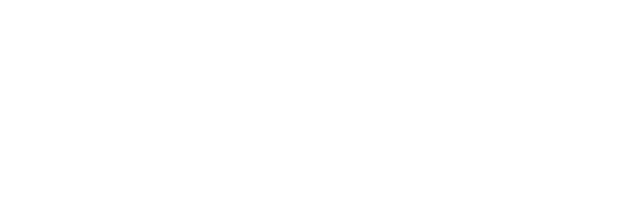### Construction of geometric figures

1. Use a protractor to accurately measure the following angles, as shown on the diagram below, and write the answers in the table provided:

1. $$\hat{B}$$

2. $$A\hat{D}B$$

3. $$D\hat{A}B$$

4. $$C\hat{D}B$$

5. reflex $$C\hat{A}B$$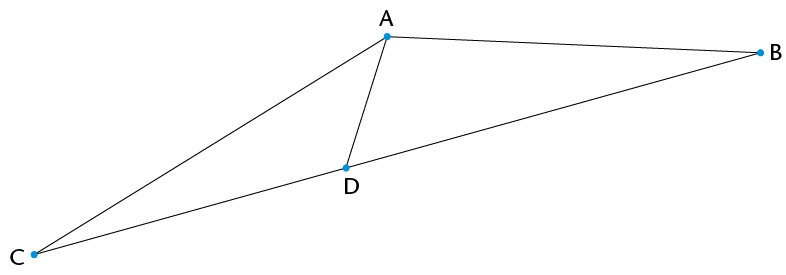Angle name Size Classification $$\hat{B}$$ $$A\hat{D}B$$ $$D\hat{A}B$$ $$C\hat{D}B$$ Reflex $$C\hat{A}B$$ Reflex
2. Construct a semi-circle with a radius of 3 cm.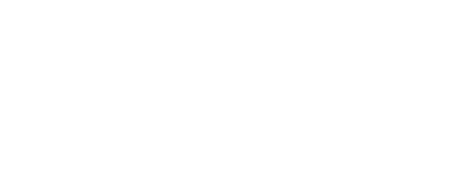3. Use a ruler and protractor to construct angles that are the given sizes. Label the angles correctly.

1. $$E\hat{F}G = 152^{\circ}$$

2. $$X\hat{Y}Z = 289^{\circ}$$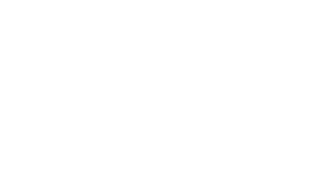4. Use circles to construct two lines, CD and EF, that are parallel to line AB below. Line CD should be above line AB, and line EF below line AB. Label both lines.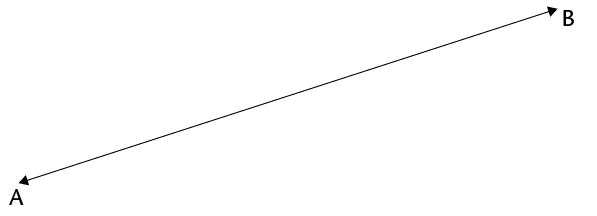5. Consider the diagram alongside.Write down the names of the pair of perpendicular lines.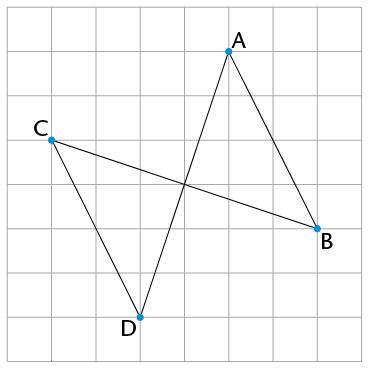### Geometry of shapes

1. Give the full name of the shape that fits the given descriptions:

1. A three-sided shape that has exactly two sides equal in length

2. A four-sided shape with both pairs of opposite sides parallel and equal in length, and with no right angles

3. A four-sided shape with only one pair of opposite sides parallel

2. What is the correct term for each of the following parts of the circle with centre B, as shown alongside?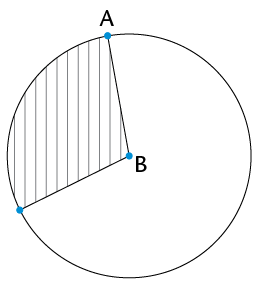1. Line AB

3. On the square grid alongside, two sides of a kite have been drawn. Use a ruler and complete the kite on the grid.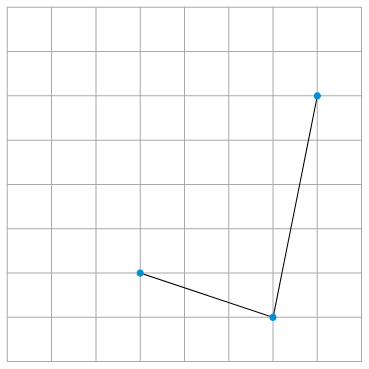4. Darrel says, "The four-sided shapes I am thinking of have at least one pair of adjacent sides equal. What are they?" Write down the names of all the shapes that fit his description.

5. DEFG is a kite, and DE = 4 cm and EF = 5,2 cm. Write down the lengths of DG and GF.

6. STUV is a rectangle. Write down the value of $$\hat{T} + \hat{V}$$. Give a reason for your answer.

7. Consider the diagram alongside.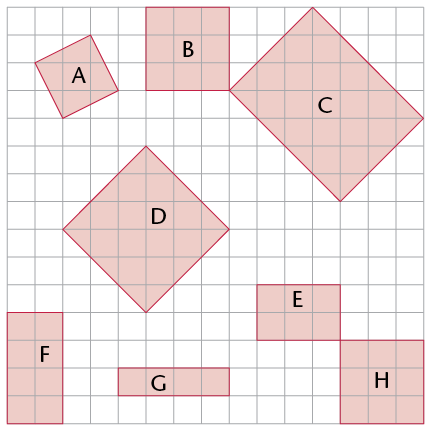1. Write down the letters of all the shape(s) that are congruent to shape B.

2. Write down the letters of all the shape(s) that are similar to shape B.

8. An isosceles triangle, LMN, has LM = 4 cm and a perimeter of 16 cm. Investigate and write down all the possible lengths of MN and LN.

9. In each case say whether the two sides are opposite sides or adjacent sides of quadrilateral DEFG.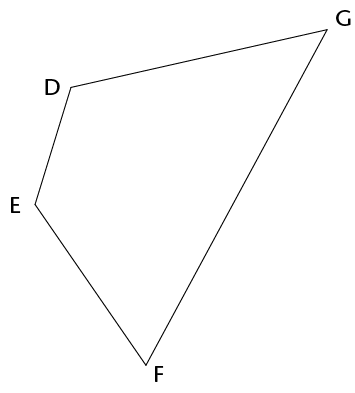1. GD and DE

2. DE and GF

## Assessment

In this section, the numbers indicated in brackets at the end of a question indicate the number of marks that this question is worth. Use this information to help you determine how much working is needed.

The total number of marks allocated to the assessment is 60.

1. Here are five one-digit cards: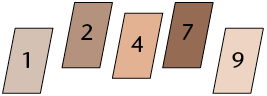Two-digit numbers can be made by placing two cards next to each other - so, for example, taking the 1 and the 2 and putting them next to each other will create the number 12 (twelve).

Choose two cards each time to make the following two-digit numbers:

1. An odd number

2. A multiple of 9

3. A factor of 126

4. A square number

2. Ayanda has a pack of cards numbered from 1 to 16. He chooses four cards at random from the pack:

• One is a factor of 39.
• Two are multiples of 4.
• Three are even.
• The total of the four numbers is more than 45, but less than 50.

Write down the values of the four numbers.

3. All 769 learners from the Sibanye Primary School are going to an athletics meeting. The school hires buses from a local company. Each bus can take only 52 passengers, and each bus has to have two teachers on board. How many buses will the school have to hire to get everyone to the meeting?

4. Calculate the total number of test wickets taken by the top four wicket takers in the Proteas' cricket history (figures correct as at June 2013):

• Shaun Pollock: 421
• Makhaya Ntini: 390
• Dale Steyn: 332
• Allan Donald: 330

5. Use prime factors to find the LCM of 42 and 18.

6. Dintle's family needs to get to Polokwane, 330 km away, by 11 a.m. If they leave at 7.40 a.m. and drive at an average speed of 100 km/h, will they reach their destination on time? Show all your working.

7. Determine the value of each of the following:

1. $$6^3 - (7^2 + 6^2)$$

2. $$(8-5)^3$$

3. $$(\sqrt{125})^2$$

4. $$12^2 - 4\sqrt{121} \div 2^2$$

5. $$3\sqrt{64}$$

1. Write down the letters of all the acute angles in the diagram.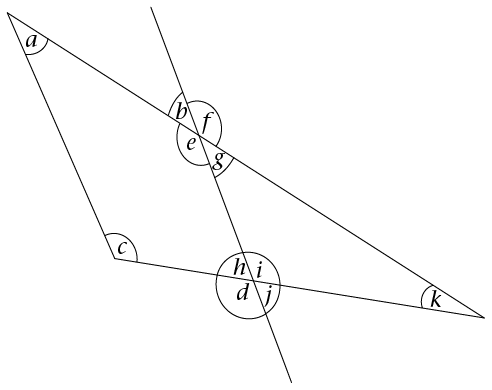2. Measure the size of angle $$d$$ in the diagram and write it down.

3. Classify angle $$d$$ according to its size.

8. Draw and correctly label angle $$K\hat{L}M = 168^{\circ}$$.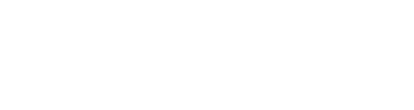9. Use your ruler and protractor to draw a line that is parallel to line segment FG drawn below, and goes through point H.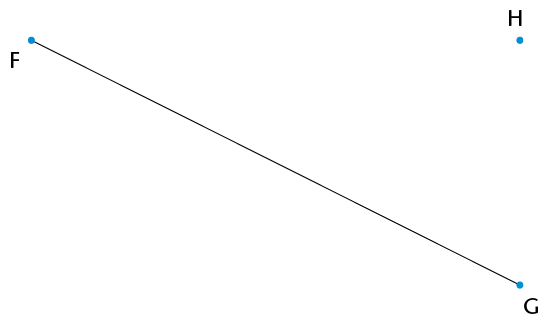10. Four circles are drawn so that they fit neatly into a square with side length of 6 cm, as shown (not to scale). Write down the radius of each circle.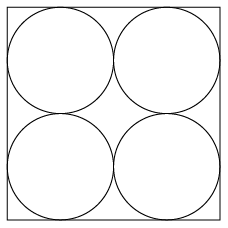1. What is the geometrical name of the shape shown on the dot grid below?

2. Draw two shapes that are similar to the shape shown, anywhere on the grid. Each shape that you draw should have a different size.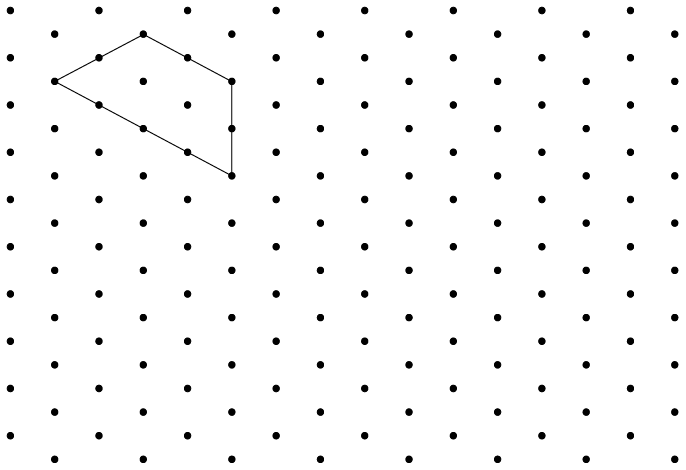11. The following diagram shows a square drawn on a dot grid. The square is divided into four triangles, namely A, B, C, and D.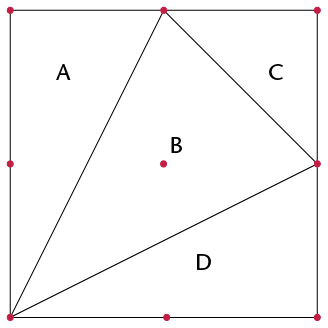1. Write down the letters of all the right-angled triangles.

2. Write down the letters of all the isosceles triangles.

3. Write down the letters of the two congruent triangles.

12. I am a quadrilateral with two pairs of opposite sides equal, no adjacent sides equal, and no right angles. What shape am I?

13. What is the special name we give to the perimeter of a circle?

14. Draw a rhombus of any size on the dot grid below. Add appropriate symbols on the diagram to show that the opposite sides of a rhombus are parallel.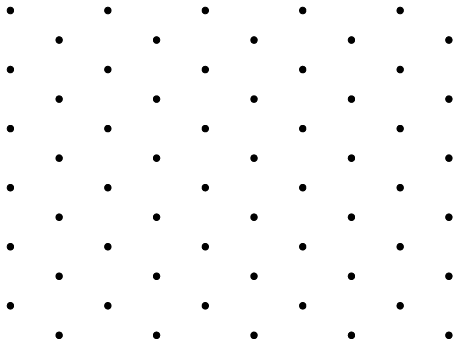15. In trapezium JKLM, JK is parallel to the opposite side.

Complete the statement:

JK ||

16. Study the following diagram: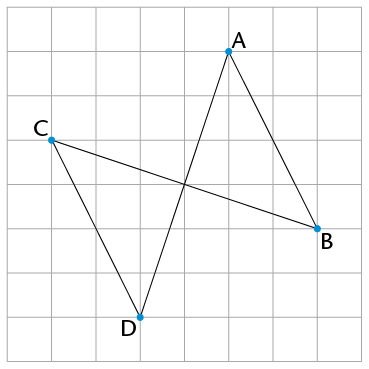Cross out the incorrect word or symbol in each set of brackets:

AD is (parallel/perpendicular) to BC. This can be shown in symbols as follows:

(AD $$\perp$$ BC / AB || BC).